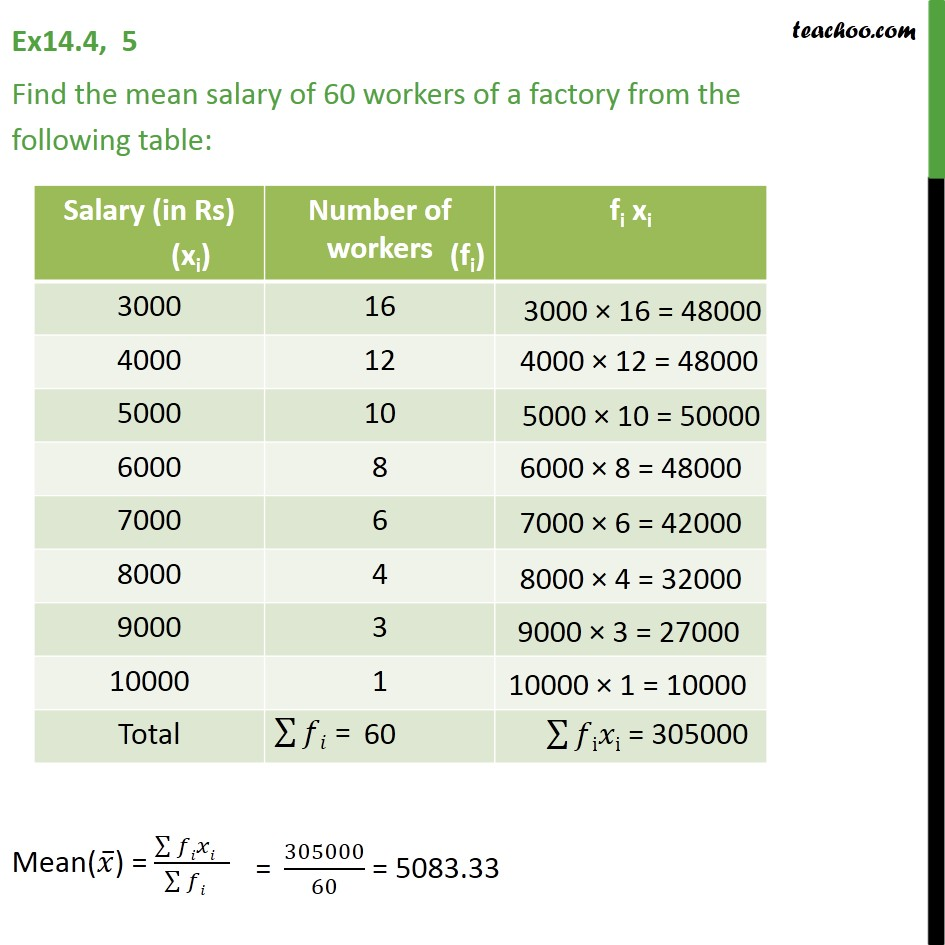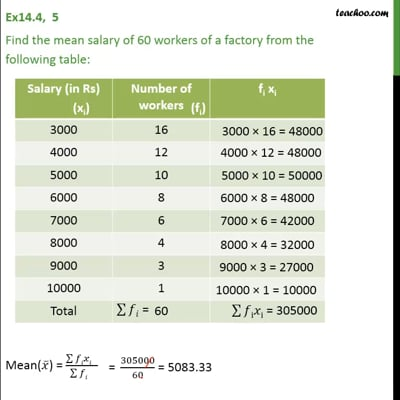Ex 14.4

Chapter 14 Class 9 Statistics (Term 1)
Serial order wiseThis video is only available for Teachoo black users

### Transcript

Ex 14.4, 5 Find the mean salary of 60 workers of a factory from the following table: Salary (in Rs) Number of workers 3000 16 4000 12 5000 10 6000 8 7000 6 8000 4 9000 3 10000 1 Total 60 3000 × 16 = 48000 4000 × 12 = 48000 5000 × 10 = 50000 6000 × 8 = 48000 7000 × 6 = 42000 8000 × 4 = 32000 9000 × 3 = 27000 10000 × 1 = 10000 ∑▒𝑓i𝑥i = 305000 Mean(𝑥 ̅) = (∑▒𝑓𝑖𝑥𝑖 " " )/(∑▒𝑓𝑖 " " ) "= " 305000/60 = 5083.33